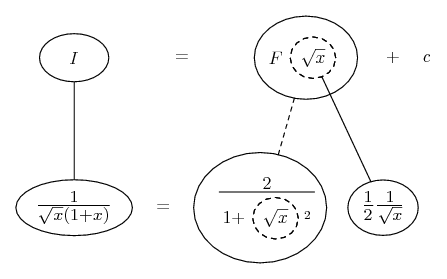# Thread: Quick integration question

1. ## Quick integration question

How do you integrate 1/( sqrt(x)*(1+x) )

Sorry for not doing the latex thing.

2. Notice it's the product of 1/stuff and 1/(1+ the square of that stuff)

(and 1/(1 + a square) is a standard integrand)

3.Originally Posted by tom@ballooncalculusNotice it's the product of 1/stuff and 1/(1+ the square of that stuff)

(and 1/(1 + a square) is a standard integrand)
there's no square

4. Just in case a picture helps...... where... is the chain rule, and you're looking for F. Straight continuous lines differentiate downwards (integrate up) with respect to x, and the straight dashed line similarly but with respect to the dashed balloon expression (which is the inner function of the composite and hence subject to the chain rule).

__________________________________________
Don't integrate - balloontegrate!
Balloon Calculus Forum
Draw balloons with LaTeX: Balloon Calculus Drawing with LaTeX and Asymptote!

5.Originally Posted by TitaniumXthere's no square
Yes there is, there's a square of $\displaystyle \sqrt x$.

#### Search Tags

integration, question, quick